# Peirce decomposition

The representation of a ring as the direct sum of subrings related to a given idempotent. For a ringcontaining an idempotent, there exist left, right and two-sided Peirce decompositions, which are defined by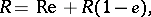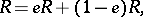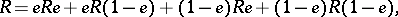respectively. Ifhas no identity, then one puts, by definition,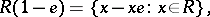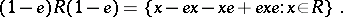The sets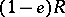and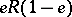are defined analogously. Therefore, in a two-sided Peirce decomposition an element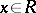can be represented asin a left decomposition as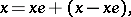and in a right decomposition asThere is also a Peirce decomposition with respect to an orthogonal system of idempotentswhere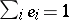:This decomposition was proposed by B. Peirce .

How to Cite This Entry:
Peirce decomposition. L.A. Skornyakov (originator), Encyclopedia of Mathematics. URL: http://encyclopediaofmath.org/index.php?title=Peirce_decomposition&oldid=15535
This text originally appeared in Encyclopedia of Mathematics - ISBN 1402006098